# Bayesian approach to analyzing differences in proportions¶

In :
from IPython.core.display import HTML
HTML('''<script>
code_show=true;
function code_toggle() {
if (code_show){
$('div.input').hide(); } else {$('div.input').show();
}
code_show = !code_show
}
$( document ).ready(code_toggle); </script> <form action="javascript:code_toggle()"><input type="submit" value="View code."></form>''')  Out: In : import csv import numpy import matplotlib.pyplot as plt plt.rcParams["figure.figsize"] = (20,5)  In : # csv file name filename_before = "Before.csv" # initializing the titles and rows list fields_before = [] rows_before = [] # reading csv file with open(filename_before, 'r') as csvfile_before: # creating a csv reader object csvreader_before = csv.reader(csvfile_before) # extracting field names through first row fields_before = next(csvreader_before) # extracting each data row one by one for row in csvreader_before: rows_before.append(row) # csv file name filename_after = "After.csv" # initializing the titles and rows list fields_after = [] rows_after = [] # reading csv file with open(filename_after, 'r') as csvfile_after: # creating a csv reader object csvreader_after = csv.reader(csvfile_after) # extracting field names through first row fields_after = next(csvreader_after) # extracting each data row one by one for row in csvreader_after: rows_after.append(row)  In : alpha = 1 # uniform prior distribution beta = 1 # uniform prior distribution x = numpy.linspace(0, 1, 1000) # possibly probabilities def distribution(n, k): numerator = numpy.math.factorial(n+alpha+beta) denominator = (numpy.math.factorial(k+alpha)*numpy.math.factorial(n-k+beta)) first_term = numerator/denominator second_term = x**(k+alpha-1.) third_term = (1-x)**(n-k+beta-1.) return first_term*second_term*third_term  ## Introduction¶ This webpage aims to do the following: • Provide a conceptual and mathematical background for Bayesian and frequentist statistics and significance testing for studying differences in proportions. • Make the case for a Bayesian approach analyzing differences in proportions. The Bayesian approach (in my view) • Has a more intuitive interpretation. • Presents information in a way that is very difficult to obfuscate or misinterpret This page was assembled to help support the analysis of survey data gathered for Carol Anne Barsody's mummy experiment. ## The Bayesian approach¶ In a Bayesian analysis, we suppose that there exists some probabibility of an event occurring which is random and unknowable, but which can be estimated via experimentation. We will never know the "true" probability for certain, but we can reduce our uncertainty in our estimate with more information from experimental trials. Let's take a simple example. Suppose that we have an unfair coin. That is, a coin for which the chances of landing heads is not necessarily 50%, but could be any value between 0 and 1. We're going to conduct an experiment to reduce our uncertainty in this coin's likelihood of landing heads. Before we start the experiment, we have no idea what the coin's heads probability is. Put another way, we assign equal probability to all possible values. This is reflected in the plot below. The x-axis shows all possible values for the coin's bias towards heads, and the y-axis shows the probability density for that value. The total area under this curve is one, since we know the real probability must be between 0 and 1, but it's flat. It's flat because we have no information that makes us suspect any one value over another. If we did have any prior information, we could make this distribution biased in the direction of our knowledge. In : def priorDemo(): plots = [] labels = [] fig, ax = plt.subplots() x = numpy.linspace(0, 1, 1000) disto = distribution(0, 0) plots.append(disto/numpy.trapz(disto, dx=1/1000.)) labels.append("N="+str(i)) count = 1 ax.plot(x, plots/numpy.trapz(plots, dx=1./1000), label="N=0 flips", linewidth=3, color='black', alpha=1) ax.fill_between(x, plots, 0, color='blue', alpha=.1) ax.legend(loc='best') plt.title("Prior distribution for coin heads probability", fontsize=24) plt.xlabel("Probability", fontsize=18) plt.ylabel("Probability density", fontsize=18) plt.xticks(fontsize=18) plt.yticks(fontsize=18) plt.ylim([0, 1.5]) plt.show() priorDemo()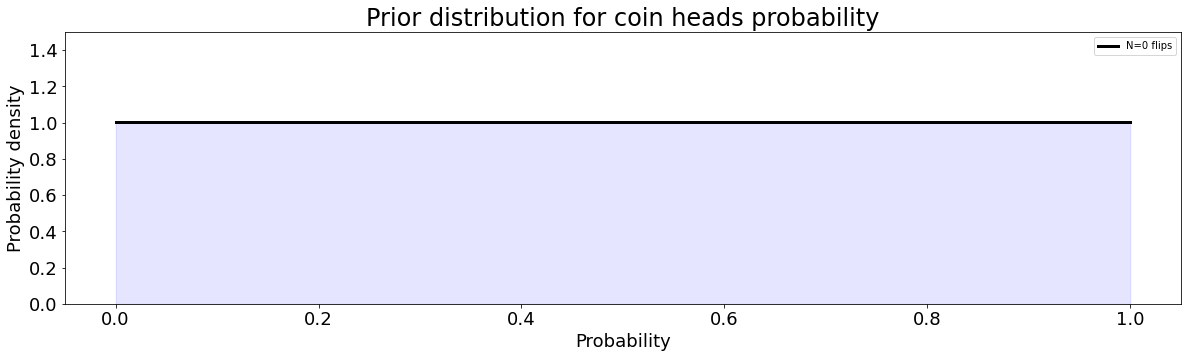We then start flipping the coin, and keeping track of how many times it lands heads. In the plot below, we've flipped the coin 10 times and counted heads 8 times. This has changed the probability density function for the heads bias. The peak of this curve is now at 0.8, but it is still quite a broad curve. This means we still have a lot of uncertainty as to the true value of the heads bias, because we haven't gathered that much information after only 10 coin flips. The curve below is the answer to the question "What is the probability of the coin's bias towards heads, given the number of heads that I've seen after 10 flips?" It makes sense that this plot is at 0 probability density (y-axis) for a heads-probability of 0 and 1 (x-axis). If the coin had zero probability of landing heads, we would never see any heads after infinite numbers of flips. Likewise, if it had a 100% chance of landing heads, we'd never see any tails. Since we've seen some of each, we know there's no chance that this coin has zero probability of landing heads, or total probability of landing heads. But the curve is nonzero for all other values! It might have a probability of landing heads of 0.4, and we just got an unusually large number of heads in our dataset! In : def N10Demo(): plots = [] labels = [] fix, ax = plt.subplots() x = numpy.linspace(0, 1, 1000) probability_heads = 0.67 dice = [] kvals = 0 for i in numpy.arange(0, 11, 1): dice.extend(numpy.random.rand(i)) if(i>0): if(dice[-1]<probability_heads): kvals += 1 disto = distribution(i, kvals) plots.append(disto/numpy.trapz(disto, dx=1/1000.)) labels.append("N="+str(i)) # plt.figure(facecolor='white') ax.plot(x, plots[-1]/numpy.trapz(plots[-1], dx=1./1000), label="N=10 flips", linewidth=3, color='red', alpha=1) ax.fill_between(x, plots[-1], 0, color='blue', alpha=.1) ax.legend(loc='best') plt.title("Likelihood for heads probability after 10 flips, landed heads "+ str(kvals)+" times", fontsize=24) plt.xlabel("Probability", fontsize=18) plt.ylabel("Probability density", fontsize=18) plt.xticks(fontsize=18) plt.yticks(fontsize=18) plt.show() N10Demo()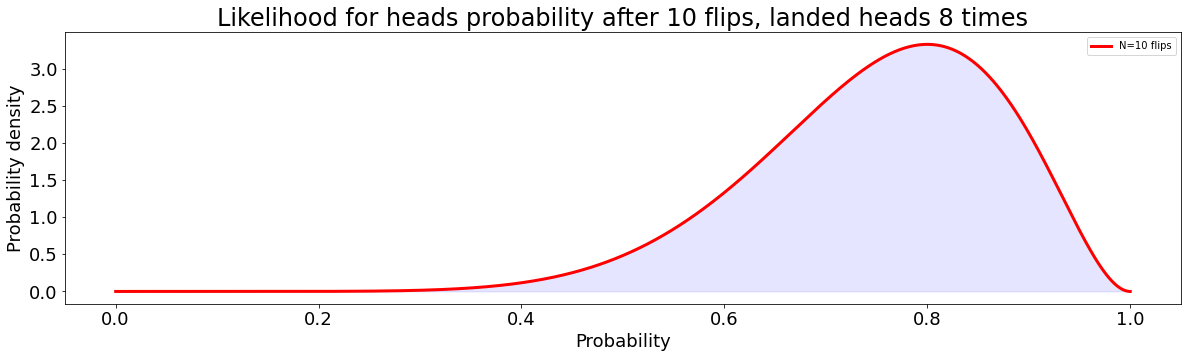The more information that we gather, the narrower and narrower this curve gets. We're adding information. In the plot below, you can see the probability density function for 0 coin flips (the flat black line), and you can see the probability density function after 1000 coin flips (the red line). I've very fainly drawn all the curves for 0-1000 flips. You can see these curves getting narrower and narrower as we learn more and more about the coin. In : def confidenceDemo(): plots = [] labels = [] probability_heads = 0.67 dice = [] kvals = 0 for i in numpy.arange(0, 1001, 1): dice.extend(numpy.random.rand(i)) if(i>0): if(dice[-1]<probability_heads): kvals += 1 disto = distribution(i, kvals) plots.append(disto/numpy.trapz(disto, dx=1/1000.)) labels.append("N="+str(i)) plt.figure(facecolor='white') count = 1 plt.plot(x, plots/numpy.trapz(plots, dx=1./1000), label="N=0", linewidth=3, color='black', alpha=1) for i in plots[1:]: plt.plot(x, i/numpy.trapz(i, dx=1./1000), linewidth=1, color='black', alpha=0.015) count += 1 plt.plot(x, plots[-1]/numpy.trapz(plots[-1], dx=1./1000), label="N=1000", linewidth=3, color='red', alpha=1) plt.legend(loc='best') plt.title("Flipping an unfair coin: increasing confidence with increasing trials", fontsize=24) plt.xlabel("Probability", fontsize=18) plt.ylabel("Probability density", fontsize=18) plt.xticks(fontsize=18) plt.yticks(fontsize=18) plt.show() confidenceDemo()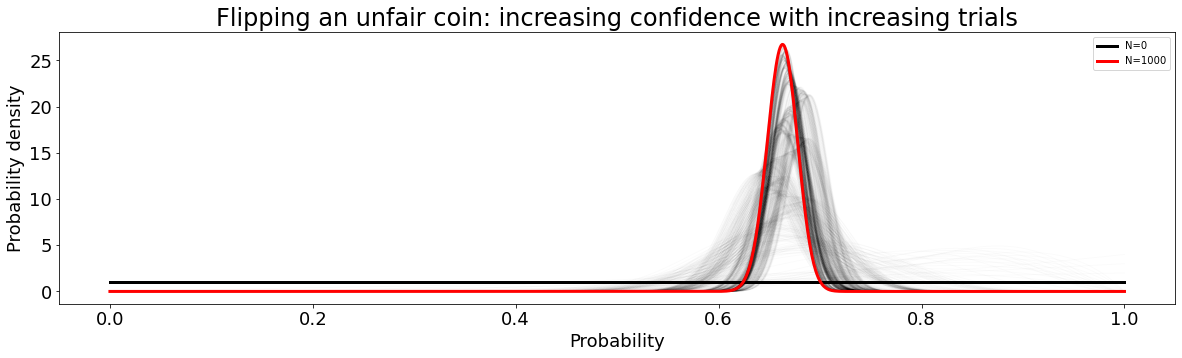The top of this curve is the maximum likelihood estimate for the unknown probability and it is part of the story. The tops of these curves will fall some distance away from the true underlying (random) probability which we are trying to estimate. In a real experiment, we can't possibly know what that probability truly is, but we can use curves like these to report credible intervals for that probability. #### Credible intervals¶ The total area under all these curves is 1. The area under a continuous section of the curve represents the probability that the underlying probability which we are trying to estimate (let's call it$p_1$) falls in that range. For example, consider the plot below for 200 flips of our unfair coin. We cannot report the probability that the coin will land heads (because we don't know for certain), but we can report our confidence that the probability is within a particular range. A 95% credible region is plotted below in red. The area under this region integrates to 0.95 (95 percent confidence) and is highlighted in blue. Remember, there is still a 5% chance that the true probability is outside this region! As we gain information and this curve gets narrower, this credible region shrinks. Note that we are numerically integrating this curve, I haven't solved it analytically. In : def intervalDemo(): kvals = 0 probability_heads = 0.67 dice = numpy.random.rand(200) for i in dice: if (i<probability_heads): kvals += 1 disto = distribution(200, kvals) disto = disto/numpy.trapz(disto, dx=(1./1000)) maxdex = list(disto).index(max(disto)) intval = 0 intdex = 1 while(intval < 0.95): intval = numpy.trapz(disto[maxdex-intdex:maxdex+intdex], dx=1./1000) intdex += 1 fig, ax = plt.subplots() ax.plot(x[0:maxdex-intdex-1], disto[0:maxdex-intdex-1], 'b') ax.plot(x[maxdex-intdex-1:maxdex+intdex], disto[maxdex-intdex-1:maxdex+intdex], 'r') ax.plot(x[maxdex+intdex:], disto[maxdex+intdex:], 'b') plt.xlabel('Probability of landing heads', fontsize=18) plt.ylabel('Probability density', fontsize=18) plt.xticks(fontsize=18) plt.yticks(fontsize=18) ax.fill_between(x[maxdex-intdex-1:maxdex+intdex], disto[maxdex-intdex-1:maxdex+intdex], 0, color='blue', alpha=.1) plt.title('95% credible interval for 200 trials of the unfair coin', fontsize=24) plt.show() intervalDemo()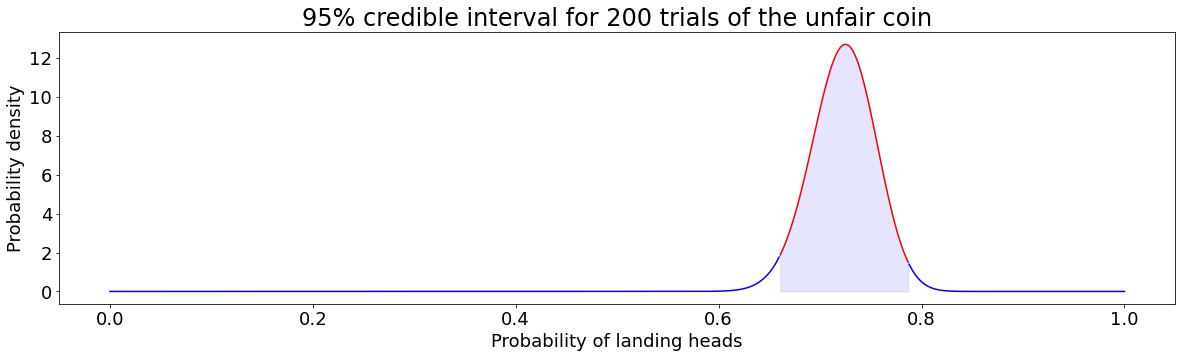#### Relationships between random variables¶ The probability density functions shown above are nice for a couple reasons. They make the quality of our information visually obvious (the flatter the curve, the less we know), and they allow for us to understand credible intervals for an unknown random variable. They can also be useful for understanding the relationship between random variables. Suppose that we have two unfair coins, each with an unknown probability of landing heads. We flip Coin A 200 times, and we flip Coin B 100 times. We count the number of times each coin lands heads, and use those counts to generate the probability density functions shown below. What can we say about the relationship between these two coins? The peak of Coin B's density function is farther right than A's, but it overlaps with that of Coin A by a considerable amount and it's flatter than Coin A's (we flipped it less times, so we have less information). How significant are these results? We can answer that question in a couple ways. In : def comparisonDemo(N): plots = [] labels = [] kvals = [90, 56] probability_heads = [0.50, 0.55] for i in range(2): disto = distribution(N[i], kvals[i]) plots.append(disto/numpy.trapz(disto, dx=1/1000.)) fig, ax = plt.subplots() ax.plot(x, plots/numpy.trapz(plots, dx=1./1000), linewidth=5, alpha=1., color='black', label='Coin A') ax.plot(x, plots/numpy.trapz(plots, dx=1./1000), linewidth=5, alpha=1., color='red', label='Coin B') plt.title("Probability density functions for two mystery coins", fontsize=24) plt.xlabel("Probability", fontsize=18) plt.ylabel("Probability density", fontsize=18) plt.xticks(fontsize=18) plt.yticks(fontsize=18) ax.legend(loc='best') plt.show() comparisonDemo([200, 100])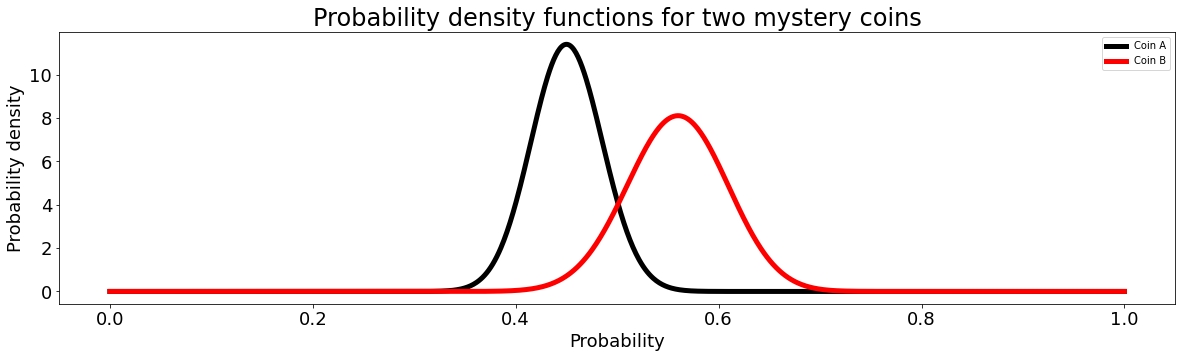#### Regions of practical equivalence¶ To a pure Bayesian, everything is probabilistic, and black-and-white decision rules go against the grain of their philosophy. The goal of a Bayesian is not dichotomous decision making, but instead the full story which is told by these posterior distributions. Even so, we can generate a decision rule based on these distributions. In the frequentist world, a parameter value is rejected if it falls outside the 95% confidence interval. We can apply a similar-but-different technique to Bayesian posterior distributions. We can't simply reject parameter values that fall outside of the 95% highest density interval for a couple reasons. First, this strategy only allows for us to reject a parameter value and never accept it. And furthermore, this strategy will eventually always reject a null value even when it's actually true. So, we need a slightly different approach. A common procedure is to establish a region of practical equivalence (ROPE). You'll sometimes hear this called by other names, including the "don't care region," "range of equivalence," "indifference zone," or "smallest effect size of interest." The notion is to establish a range of parameter values that are practically equivalent to the null value. "Practical equivalence" depends on the particular situation. In the case of the coins, we might say that heads biases that are within, say, 0.01 (just making up a number) are practically the same. In a clinical medicine environment, this number may be smaller. For other applications, it may be larger. Our decision rule is then this: If the 95% highest density interval (blue-shaded region above) falls entirely outside the ROPE associated with the null value, then we reject the null value (not the entire ROPE interval). If the 95% HDI falls entirely inside the ROPE, then we accept the ROPE'd value for practical purposes. And otherwise we remain undecided. This can be used to compare posterior distributions. #### Using ROPE's to compare distributions¶ Does there exist a null value with a non-zero ROPE which we accept for the variable described by one distribution, and reject for the variable described by the other? If yes, then the decision rule accepts that the random variables are not practically the same. Otherwise, the decision rule remains undecided until the ROPE is wide enough to include the 95% confidence intervals for both distributions. In the example below, the decision rule remains undecided about the difference in heads probabilities between the two coins, until the ROPE stretches from the bottom of the HDI for Coin A all the way to the top of the HDI for Coin B (from 0.38 to 0.656). So if our null value were 0.518 with a region of practical equivalence from 0.38 to 0.656, then we would accept the ROPE'd value for both coins. In other words, we'd say that all the 95% most credible values for the heads bias for both coins are practically equivalent to the null value. Now, in this case, that region of practical equivalence is way too large to be practical (probably). But it illustrates the point. In : def bayesSigDemo(N): plots = [] labels = [] kvals = [90, 56] probability_heads = [0.50, 0.55] for i in range(2): disto = distribution(N[i], kvals[i]) plots.append(disto/numpy.trapz(disto, dx=1/1000.)) maxdex = list(plots).index(max(plots)) intdex = 1 intval = 0 while(intval < 0.95): intval = numpy.trapz(plots[maxdex-intdex:maxdex+intdex+1], dx=1./1000) intdex += 1 maxdex2 = list(plots).index(max(plots)) intdex4 = 1 intval = 0 while(intval < 0.95): intval = numpy.trapz(plots[maxdex2-intdex4:maxdex2+intdex4+1], dx=1./1000) intdex4 += 1 lower = ((maxdex2-intdex4) - (maxdex+intdex+1))/1000. upper = ((maxdex2+intdex4+1) - (maxdex-intdex))/1000. fig, ax = plt.subplots() ax.plot(x, plots/numpy.trapz(plots, dx=1./1000), linewidth=5, alpha=1., color='black', label='Coin A') ax.fill_between(x[maxdex-intdex:maxdex+intdex+1], plots[maxdex-intdex:maxdex+intdex+1], 0, color='black', alpha=.1) ax.plot(x, plots/numpy.trapz(plots, dx=1./1000), linewidth=5, alpha=1., color='red', label='Coin B') ax.fill_between(x[maxdex2-intdex4:maxdex2+intdex4+1], plots[maxdex2-intdex4:maxdex2+intdex4+1], 0, color='red', alpha=.1) plt.title("Highest density intervals for two mystery coins", fontsize=24) plt.xlabel("Probability", fontsize=18) plt.ylabel("Probability density", fontsize=18) plt.xticks(fontsize=18) plt.yticks(fontsize=18) ax.legend(loc='best') plt.show() bayesSigDemo([200, 100])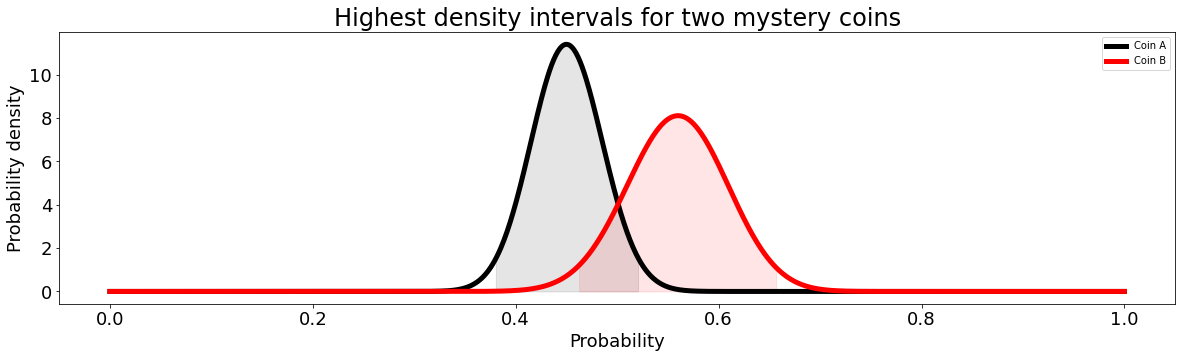#### How do we generate these distributions from a dataset?¶ We obtain these probability density functions by applying Bayes' Rule, shown below. In this equation,$p(k|x)$is the probability of seeing$k$heads given a particular probability,$x$, of the coin landing heads.$p(x)$is the prior distribution on the coin's probability of landing heads (did we have any information about this before the experiment started?), and$p(k)$is the marginal probability of seeing$kheads. \begin{align} p(x|k) &= \frac{p(k|x)p(x)}{p(k)} \end{align} Each coin flip has a probabilityx$of being heads, and a probability$(1-x)$of being tails. Thus, each flip is a Bernoulli random trial. A sequence of such trials is a Bernoulli process with a probability mass function given by a binomial distribution, shown below. This equation tells us the probability of seeing$k$heads, given a particular probability that each flip lands heads,$x. \begin{align} p(k|x) &= \frac{n!}{k!(n-k)!}x^k (1-x)^{n-k} \end{align} Just to give an example, here is the probability of seeing 0-10 heads after 10 coin flips, if the coin has a 50% chance of being heads for each flip. In other words, this plot showsp(k|x)$for each possible value of$k$(0-10),$n=10$flips, and$x=0.5$probability of landing heads. In : def binomial(n, k, x): probs = [] for i in k: numerator = numpy.math.factorial(n) denominator = (numpy.math.factorial(i)*numpy.math.factorial(n-i)) first_term = numerator/denominator second_term = x**(i) third_term = (1-x)**(n-i) probs.extend([first_term*second_term*third_term]) fig, ax = plt.subplots() ax.bar(numpy.arange(0, n+1, 1), probs) plt.title("Probability of 0-"+str(n)+" heads after "+str(n)+" coinflips, unbiased coin", fontsize=24) plt.xlabel("Number of heads", fontsize=18) plt.ylabel("Probability", fontsize=18) plt.xticks(numpy.arange(0, n+1, 1), fontsize=18) plt.yticks(fontsize=18) plt.show() binomial(10, numpy.arange(0, 11, 1), 0.5)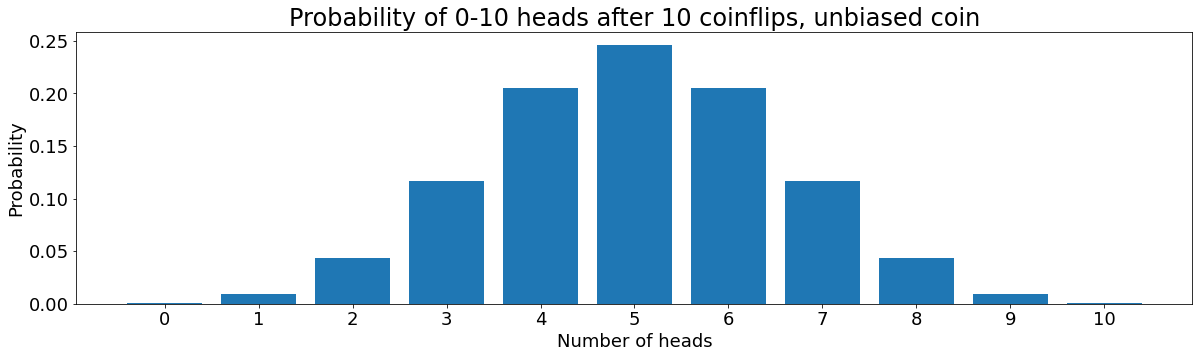Let's now think about$p(x)$, which is the prior distribution on$x$. One might choose any one of number of distributions to represent the prior. However, the beta distribution is the conjugate prior for the binomial distribution. So, by choosing to describe$p(x)$with a beta distribution, we know that$p(x|k)will also be a beta function. In other words, we're making a strategic choice so that our problem is actually solvable. \begin{align} p(x) = \frac{\Gamma(\alpha + \beta)}{\Gamma(\alpha)\Gamma(\beta)}x^{\alpha-1}(1-x)^{\beta-1} \end{align} For different choices of the parameters\alpha$and$\beta$, this function has different shapes. If we let$\alpha=\beta=1$, we get a uniform distribution. That is, we don't assume any prior knowledge on the value of$x$. This prior distribution looks like the one shown below. In : def priorDemo(): plots = [] labels = [] fig, ax = plt.subplots() x = numpy.linspace(0, 1, 1000) disto = distribution(0, 0) plots.append(disto/numpy.trapz(disto, dx=1/1000.)) labels.append("N="+str(i)) count = 1 ax.plot(x, plots/numpy.trapz(plots, dx=1./1000), label="N=0 flips", linewidth=3, color='black', alpha=1) ax.fill_between(x, plots, 0, color='blue', alpha=.1) ax.legend(loc='best') plt.title("Prior distribution for coin heads probability", fontsize=24) plt.xlabel("Probability", fontsize=18) plt.ylabel("Probability density", fontsize=18) plt.xticks(fontsize=18) plt.yticks(fontsize=18) plt.ylim([0, 1.5]) plt.show() priorDemo()Lastly, we need$p(k)$, the marginal probability of seeing$kheads. This is calculable using the chain rule and has a known solution: \begin{align} p(k) &= \int_k p(k|x)p(x)dx\\ &= \frac{n!}{k!(n-k)!} \cdot \frac{\Gamma(\alpha + \beta)}{\Gamma(\alpha)\Gamma(\beta)}\cdot \frac{\Gamma(k+\alpha)\Gamma(n-k+\beta)}{\Gamma(n+\alpha+\beta)} \end{align} Substituting everything in, we get: \begin{align} p(x|k) &= \frac{p(k|x)p(x)}{p(k)}\\ &= \frac{\Gamma(n+\alpha+\beta)}{\Gamma(k+\alpha)\Gamma(n-k+\beta)} x^{k+\alpha-1}(1-x)^{n-k+\beta-1} \end{align} For an observed number of heads,k$after a number of coinflips$n$, we can substitute in any probability$x$to learn the likelihood that the coin's probability landing heads is$x$.$\alpha$and$\beta$are both set to 1 for a uniform prior distribution. ## The frequentist approach¶ The frequentist statistician treats the underlying (and unknown) probability as a fixed value, and the confidence ranges as random. Unlike a credible range, for which the interpretation is "there is a 95% chance that the random number is in this range," the interpretation for a confidence interval is "the value of the unknown number is inside 95% of confidence intervals computed from different datasets." #### The frequentist approach to significance testing¶ For each coin, there is a (true and unknown) probability of landing heads. Let's call these probabilities$\pi_1$and$\pi_2$. In an effort to estimate these values, we've flipped the coins a number of times and generated and counted the number of times that each landed heads. We'll call these counts$k_1$and$k_2$. The random variables$k_1$and$k_2$follow a binomial distribution with means at$N_1 \pi_1$and$N_2 \pi_2$, and with variances$N_1 \pi_1 (1-\pi_1)$and$N_2 \pi_2 (1-\pi_2)$. For a sufficiently large sample size$N, these binomial distributions are well approximated by normal distributions. \begin{align} k_1 &\sim \mathcal{N}\left(N_1 \pi_1, N_1 \pi_1 (1-\pi_1)\right)\\ k_2 &\sim \mathcal{N}\left(N_2 \pi_1, N_2 \pi_2 (1-\pi_2)\right) \end{align} The quality of these approximations depends both on the number of samples,N$, and on the probability of landing heads,$\pi_1$. In the plots below, you can see the comparisons between a binomial distribution and a Gaussian distribution for increasing$N$and$\pi=0.5$. A rule of thumb is that the approximation holds if$N\pi(1-\pi) > 5$. In : def binomialDemo(N, p): k = numpy.arange(0, N) result = [] for i in k: first_term = (numpy.math.factorial(N)/ (numpy.math.factorial(i)*numpy.math.factorial(N-i))) second_term = (p**(float(i)))*((1-p)**(float(N-i))) result.extend([first_term * second_term]) mean = float(list(result).index(max(result))) p = float(mean)/N sigma = numpy.sqrt(N*p*(1-p)) x = numpy.linspace(0, N, 1000) gaussian = ((1./(sigma*numpy.sqrt(2*numpy.pi))) * numpy.exp(-0.5*((x - mean)/sigma)**2.)) plt.plot(result, label='binomial distribution') plt.plot(x, gaussian, label='gaussian distribution') plt.xlabel("Number of heads counted", fontsize=18) plt.ylabel("Probability density, p="+str(p), fontsize=18) plt.title("Total number of flips: "+str(N), fontsize=24) plt.xticks(fontsize=18) plt.yticks(fontsize=18) plt.legend(loc='best') plt.show() binomialDemo(10, .5) binomialDemo(50, .5) binomialDemo(100, .5)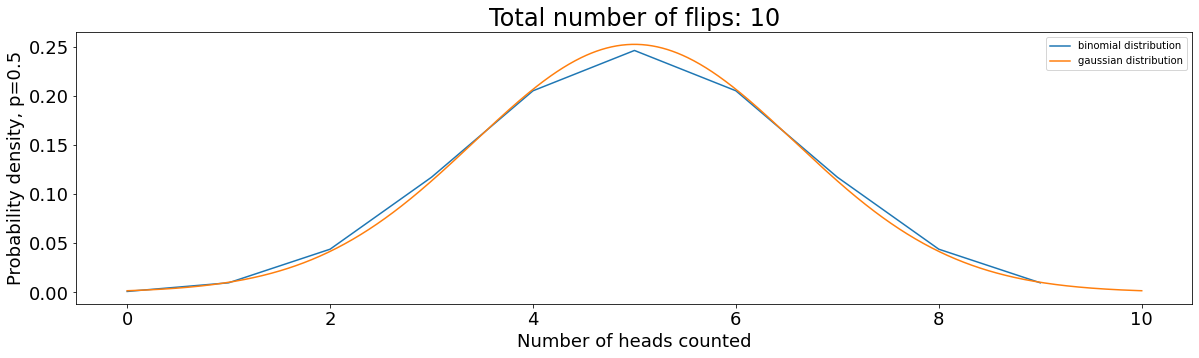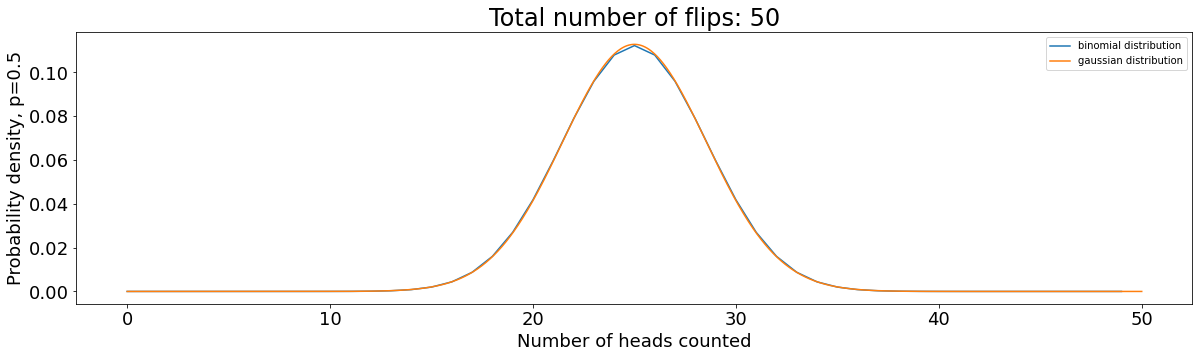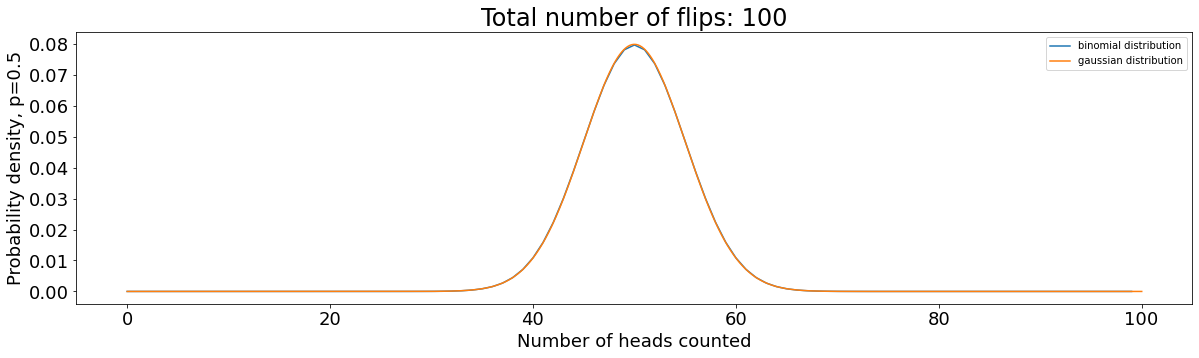Linear combinations and transformations of normally distributed random variables are also normally distributed! This implies that our sample proportions,$p_1=\frac{k_1}{N_1}$and$p_2=\frac{k_2}{N_2}, are also normally distributed. \begin{align} p_1 &\sim \mathcal{N}\left(\pi_1, \frac{\pi_1(1-\pi_1)}{N_1}\right)\\ p_2 &\sim \mathcal{N}\left(\pi_2, \frac{\pi_2(1-\pi_2)}{N_2}\right) \end{align} We can solve for the distribution which describes the difference betweenp_1$and$p_2. We just subtract the means and add the variances, since we're dealing with normal distributions. \begin{align} p_1-p_2 \sim \mathcal{N} \left(\pi_1 - \pi_2, \frac{\pi_1(1-\pi_1)}{N_1} + \frac{\pi_2(1-\pi_2)}{N_2}\right) \end{align} From this distribution which describes the random variable which is the difference in our sample proportions, we can generate a normalized test statistic. If we substract(\pi_1 - \pi_2)from the above normal distribution and divide by the square root of the variance, then we are left with a normal distribution with mean 0 and standard deviation of 1! \begin{align} z &= \frac{(p_1 - p_2) - (\pi_1 - \pi_2)}{\sqrt{\frac{\pi_1(1-\pi_1)}{N_1} + \frac{\pi_2(1-\pi_2)}{N_2}}} \sim \mathcal{N}(0, 1) \end{align} The denominator in the above term is the standard deviation ofp_1 - p_2$. Because we don't know$\pi_1$and$\pi_2, we can't know the true value of this denominator. So! We use an estimate of the standard error - the standard deviation of its sampling statistic. \begin{align} z &= \frac{(p_1 - p_2) - (\pi_1 - \pi_2)}{\sqrt{\frac{p_1(1-p_1)}{N_1} + \frac{p_2(1-p_2)}{N_2}}} \sim{N}(0, 1) \end{align} We can now decide on the confidence range that we'd like to determine for the random variablez. We'll demand 95% confidence, or two standard deviations. \begin{align} -2 \leq z = \frac{(p_1 - p_2) - (\pi_1 - \pi_2)}{\sqrt{\frac{p_1(1-p_1)}{N_1} + \frac{p_2(1-p_2)}{N_2}}} \leq 2 \end{align} Multiply thru by the denominator: \begin{align} -2\sqrt{\frac{p_1(1-p_1)}{N_1} + \frac{p_2(1-p_2)}{N_2}} \leq (p_1 - p_2) - (\pi_1 - \pi_2) \leq 2\sqrt{\frac{p_1(1-p_1)}{N_1} + \frac{p_2(1-p_2)}{N_2}} \end{align} Multiply by -1, and add(p_1 - p_2): \begin{align} (p_1 - p_2)-2\sqrt{\frac{p_1(1-p_1)}{N_1} + \frac{p_2(1-p_2)}{N_2}} \leq (\pi_1 - \pi_2) \leq (p_1 - p_2) + 2\sqrt{\frac{p_1(1-p_1)}{N_1} + \frac{p_2(1-p_2)}{N_2}} \end{align} If the above range includes 0, then the difference in proportions is insignificant for our chosen level of significance. Let's apply this analysis to our red and black coin flip curves from the previous section. We flipped Coin A 200 times, and got 105 heads. We flipped Coin B 100 times and got 60 heads. What is the 95% confidence interval for the difference in the probability of heads for Coins A and B? \begin{align} p_1 &= \frac{k_1}{N_1} = \frac{56}{100} = 0.56\\ p_2 &= \frac{k_2}{N_2} = \frac{90}{200} = 0.45 \end{align} Substituting these into the expression above yields a 95% confidence interval for the difference in coin probabilities of: In : def confidenceInterval(k1, N1, k2, N2, stdvs): p1 = float(k1)/N1 p2 = float(k2)/N2 stderr = numpy.sqrt(((p1*(1-p1))/N1) + ((p2*(1-p2))/N2)) lower = (p1-p2) - stdvs*stderr upper = (p1-p2) + stdvs*stderr print("["+str(lower)+", "+str(upper)+"]") confidenceInterval(56, 100, 90, 200, 2.)  [-0.01167990795525771, 0.23167990795525778]  Because this confidence interval contains 0, the results in this example are not significant. ## Comparing approaches¶ Which approach to confidence testing is better? It's a matter of taste, but here are the differences: • Philosophically, Bayesians treat the underlying probability as a random variable and the credible region boundaries as fixed. Frequentists treat the underlying probability as fixed, and the confidence region as a random variable. • Bayesian credible intervals require a prior distribution for the underlying random variable (which could be a uniform distribution, but needn't be). Frequentist confidence intervals do not. • The confidence interval: random samples from the same population and with the same sample sizes would yield confidence intervals that contain the true and unknown value 95% (or whatever threshold was enforced) of the time. Or in other words, a confidence interval computed from some data will include the true value of the unknown parameter 95% of the time. • The credible interval: given the observed data, the unknown value has a 95% (or whatever threshold was enforced) probability of falling in this range. In other words, there is a 95% chance that the true value of the parameter is in this interval. I prefer the Bayesian approach. For these sorts of analyses, I think their interpretation is more intuititive, and it does not require that we apply any assumptions, approximations, or rules of thumb. I think that the posterior distributions illustrate knowledge in a way that is difficult to obfuscate or misinterpret. ## Code for applying Bayesian analysis to survey data¶ Click "view code" at the top of the webpage for a Python function that generates probability density functions and credible intervals, given a list ofN$and$k\$ values for multiple populations.

In :
def compare(nlist, klist, labels, x_label):

fig, ax = plt.subplots()
fig.facecolor='white'
x = numpy.linspace(0, 1, 1000)

colors = ['black', 'red', 'blue', 'green', 'magenta', 'orange', 'yellow']

for i in range(len(nlist)):
disto = distribution(nlist[i], klist[i])
disto = disto/numpy.trapz(disto, dx=1/1000.)

maxdex = list(disto).index(max(disto))

intdex_95 = 1
intval = 0
while(intval < 0.95):
intval = numpy.trapz(disto[max(0, maxdex-intdex_95):min(maxdex+intdex_95+1, 1000)], dx=1./1000)
intdex_95 += 1

intdex_97 = 1
intval = 0
while(intval < 0.9747):
intval = numpy.trapz(disto[max(0, maxdex-intdex_97):min(maxdex+intdex_97+1, 1000)], dx=1./1000)
intdex_97 += 1

print(colors[i]+" 95.00:\t ["+
str((max(0, maxdex-intdex_95))/1000.)+', '+
str((min(maxdex+intdex_95+1, 1000))/1000.)+']')
print(colors[i]+" 97.47:\t ["+
str((max(0, maxdex-intdex_97))/1000.)+', '+
str((min(maxdex+intdex_97+1, 1000))/1000.)+']\n')

ax.plot(x, disto/numpy.trapz(disto, dx=1./1000),
linewidth=5, alpha=1., color=colors[i], label=labels[i])
ax.fill_between(x[maxdex-intdex_95:maxdex+intdex_95+1],
disto[maxdex-intdex_95:maxdex+intdex_95+1],
0, color=colors[i], alpha=.1)

plt.xlabel(x_label, fontsize=18)
plt.ylabel("Probability density", fontsize=18)
plt.xticks(numpy.linspace(0, 1, 21), fontsize=18, rotation=45)
plt.yticks(fontsize=18)
plt.grid()
fig.patch.set_facecolor('white')
ax.legend(loc='best', fontsize=18)
plt.savefig('plot.png', dpi=300, bbox_inches='tight')
plt.show()